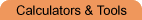Home    |    Teacher    |    Parents    |    Glossary    |    About UsEmail this page to a friendSelect Tool· Calculators · Converters · Equation Solvers · GraphersResources· Cool Tools · Formulas & Tables · References · Test Preparation · Study Tips · Wonders of MathSearchMath.com Online Calculators

Basic Calculator - quick and simple

Scientific Calculator - full featured: logs, trig, hex

Square Root - find square root of any number

Circle - find area, radius, perimeter

Prime Number - is it prime?

Quadratic Equations - input a, b, c and find roots

Percents - 3 ways to calculate percentages, percent of, etc.

 Alert! If your teacher doesn't allow calculators for homework, that means online ones, too. OK? OK.

(see note on precision)

Trigonometry

Probability

Finance

 Related Resources

Decimal to Fraction Calculator - (Elementary/Middle School)
Turns any decimal into an equivalent or approximate fraction.

Roman Numeral Calculator - Just like Caesar used to have! Fun to use.

Student Loan Calculators - How much will your student loan payments be?

Multiplying Polynomials Calculator - (Middle School/High School)
Put in any two polynomials and this calculator will multiply them together and show the steps to get the answer.

Integral Calculator - (High School/College) Integrate functions instantly.

Vector Calculator - (High School/College) Graphically add two vectors together. Shows numerical results.

The MathServ Calculus Toolkit - (High School/College) Many algebra and calculus tools. Polynomials, functions, graphing, derivatives, limits, integration and more. Also a separate differential equation toolkit. (Vanderbilt University)

Precision and accuracy. Please be aware that there are certain limitations to all web-based calculators. The arithmetic used can lead to errors in some calculations when the numbers get very big or very small. If your work depends on being absolutely, positively accurate to the last decimal place - use a real calculator!